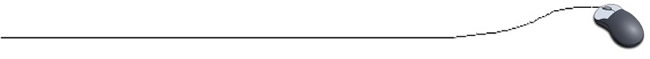Binary Search

Do you remember playing the game "Guess a Number", where the responses to the statement "I am thinking of a number between 1 and 100" are "Too High", "Too Low", or "You Got It!"?  A strategy that is often used when playing this game is to divide the intervals between the guess and the ends of the range in half.  This strategy helps you to quickly narrow in on the desired number.

When searching an array, the binary search process utilizes this same concept of splitting intervals in half as a means of finding the "key" value as quickly as possible.

If the array that contains your data is in order (ascending or descending), you can search for the key item much more quickly by using a binary search algorithm ("divide and conquer").

Consider the following array of integers:

 13 24 34 46 52 63 77 89 91 100 num num num num num num num num num num

We will be searching for the integer 77:

• First, find the middle of the array by adding the array subscript of the first value to the subscript of the last value and dividing by two:  (0 + 9) / 2 = 4    Integer division is used to arrive at the 4th subscript as the middle. (The actual mathematical middle would be between the 4th and 5th subscripts, but we must work with integer subscripts.)

• The 4th subscript holds the integer 52, which comes before 77.  We know that 77 will be in that portion of the array to the right of 52.  We now find the middle of the right portion of the array by using the same approach.  (5 + 9) / 2 = 7

• The 7th subscript holds the integer 89, which comes after 77.  Now find the middle of the portion of the array to the right of 52, but to the left of 89. (5 + 6) / 2 = 5

• The 5th subscript holds the integer 63, which comes before 77, so we subdivide again
(6 + 6) / 2 = 6   and the 6th subscript holds the integer 77.

import java.util.Scanner;

public class BinarySearchExample
{
public static void main(String[] args)
{
int key = 77;
int[ ] num = new int ;

// Fill the array
for (int i = 0; i < 10; i++)
{
System.out.println("Enter integer: ");
}

// The binary search method
binarySearch (num, 0, 9, key);
}

 Binary search method: binarySearch (num, 0, 9, key);  The arguments/parameters are: • array - the name of a sorted array • lowerbound - subscript (index)     of 1st element to search, array • upperbound - subscript (index)     of last element to search, array • key - item we wish to find.
//Binary Search Method
// Method accepts a pre-sorted array,
// subscript of starting element for search,
// subscript of ending element for search,
// and key number for which we are searching.

public static void binarySearch(int[ ] array, int lowerbound, int upperbound, int key)
{
int position;
int comparisonCount = 1;
// counting the number of comparisons (optional)

// To start, find the subscript of the middle position.
position = ( lowerbound + upperbound) / 2;

while((array[position] != key) && (lowerbound <= upperbound))
{
comparisonCount++;
if (array[position] > key)
// If the number is > key, ..
{
// decrease position by one.
upperbound = position - 1;
}
else
{
lowerbound = position + 1;
// Else, increase position by one.
}
position = (lowerbound + upperbound) / 2;
}
if (lowerbound <= upperbound)
{
System.out.println("The number was found in array subscript " + position);
System.out.println("The binary search found the number after " +
comparisonCount + " comparisons.");

// printing the number of comparisons is optional
}
else
{

System.out.println("Sorry, the number is not in this array.");
System.out.print(" The binary search made "+comparisonCount +" comparisons.");
}
}

}

 OUTPUT (after data from the table has been entered, searching for 77): The number was found in array subscript 6 The binary search found the number after 4 comparisons.

This method simply prints the result of the search.  You may wish to return the result of the binary search to be used in further investigations.  To do so, return the "position" if the value is found and return a negative number (for example) if the result is not found.